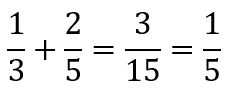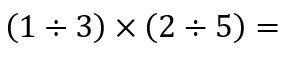# Operations on Fractions

### A tale of carts and horses.

My child is in fifth grade. Last Friday, we received the second volume of his mathematics workbook. It starts with multiplication of fractions; the next chapter is division of fractions. Addition (and subtraction) of fractions with different denominators is in the first volume.

I believe this order persists partly by conceptual understanding: What it means to add fractions together is simpler to understand than what it means to multiply them. At the same time, however, the ordering is hardly new; Milne’s 1895 mechanics-heavy primer “Elements of Arithmetic”, for instance, puts addition before multiplication.

Mechanically, multiplication of fractions is far easier than addition. Even division is mechanically easier. Indeed, from a mechanical standpoint, adding fractions requires multiplying fractions, but not vice versa.

Overall, I agree with the philosophy that we ought to be teaching conceptual understanding of mathematics instead of plain algorithms. However, I’m concerned that ordering operations on fractions parallel to operations on integers may be contributing to the confusion students have over fractions.

Today, I worked with my child on multiplying fractions. We started with an addition problem:

He was confident in his answer:

I asked him to explain his reasoning, which he did: You need to multiply the denominators and add the numerators.

Both of these steps are correct according to the standard algorithm, but he’s also missing a crucial middle step. This strikes me as more sophisticated then the more common error, which is just “add the numerators, add the denominators”. But it still results in an error, which my child saw as soon as I pointed this out:We can’t add a positive value to two-fifths and get one-fifth.

So we discussed the proper way to add fractions with unlike denominators, after which he remembered having gone through that in school.

The next step: Multiplication of fractions.

I wrote this down:

and asked him how he’d approach it.

He showed a high degree of anxiety, and then suggested somehow dividing the second fraction from the first one. I asked him to elaborate, and he figured that because addition involves multiplication, multiplication must have to involve division.

This is a reasonable conclusion, and based on how my older students approach this sort of problem, it might be a common one.

I asked him how many operators he saw there, and he told me: One. Very confident, very firm. Multiplication. I asked him if there were any other operators. No. Just the one.

I asked him to circle all of the mathematical objects he saw, and he drew four circles: Each fraction, the multiplication, and the equality. I told him I saw eight, not four, and he looked again and acknowledged that maybe he could see that.

Consider this, which is the next thing I wrote:The same thing as before. Honest.

A key understanding needed especially at higher level of Algebra is that that vinculum (the fraction bar) is a grouping symbol that represents division. I have noticed that few of my high school students have internalized this understanding. In most cases, they claim I’m the first teacher (in Algebra II) to have even pointed it out (though I have no idea how true that is).

A conceptual understanding of what a fractional value represents is important to a robust number sense. This can compete with an equally important understanding that a fractional form is a way of representing division. Milne (1895: 150) expresses this plainly:

“A fraction may be regarded as expressing unexecuted division.”

The obelus (÷) is one of the first proto-algebraic symbols elementary students see, but I’m concerned that this is rarely pointed out to them. What I mean by “proto-algebraic” is that the symbol shows precisely how to convert an elementary division expression into a fraction: 1÷2 = ½, for instance. The obelus visually says, “The expression before me is the numerator, the expression after me is the denominator — hence my two dots — and then you draw a vinculum between them.”

So those dots are place-holders, in the same way that squares are placeholders in early grades and x’s are place-holders in later graders.

Sowhat’s really going on, mechanically, when we add fractions with unlike denominators?

Conceptually, we can’t add fractions with unlike denominators. It doesn’t make sense. We need to convert our fractions to having the same denominators, and then add those. Specifically, to use the first example:

But why does this work?

It relies on the concepts that x/x=1 (for all non-zero values), and x*1=x. So what we’re doing in the second and third steps is rewriting each of the addends into equivalent expressions.

If we don’t overtly mention it, students might not even notice that they’re multiplying fractions.

There are three levels of operations in basic arithmetic: Addition, multiplication, and exponentiation. Prior to Calculus, students may only see variants on these and the trigonometric functions as the complete set of things we can do with numbers.

Because fractions inherently involve division, multiplication and division of fractions are both mechanically easier than addition, particularly when the denominators are different.

Even when the denominators are the same, though, adding fractions has a component of mystery: Why don’t we do anything to the denominator? Addition only functions on the numerators; we leave the denominator the same.

When multiplying and dividing fractions, we do something mathematical with all four of the numbers. In multiplication, we multiply the tops and multiply the bottoms. Mechanically speaking, it should only take a matter of minutes to teach someone how to do that. (What it “means” to multiply two fractions is a different matter.)

Once I had talked through all of this but without explicitly telling my child how to multiply, I wrote this down:

I told him to look at the first three examples and then do the last two. He took a minute or so, then wrote down 12/63 and 2/15.

I didn’t have him simplify the fractions. That was a separate discussion. 6/56 and 3/28 are the same value; suggesting that 6/56 is incorrect or incomplete for the second example is, in my view, flat-out wrong if the instruction is “multiply the fractions”.

I then told him that I wasn’t going to teach him how to divide fractions. I was going to do the same thing: Give him some examples and have him figure out how to do it himself. So I wrote:

This took him more time and he was clearly less comfortable, but after noting the pattern, he did write 5/4 (correcting himself from 4/5) and 12/7 for the last two.

Conceptually, I’m confident that he has no idea what it means to divide a fraction by another fraction. On teacher boards, I’ve seen elementary teachers building contrived scenarios in which someone would need to divide a fraction by a fraction.

I spend most of my time living in the realm of Algebra II and above. There is a purpose to conceptual understanding of some of the topics I cover, but I don’t know what it means conceptually to divide a polynomial by another polynomial, or what it means conceptually to take the sine of a sine.

I believe there’s a sweet spot between teaching concepts and teaching mechanics, and I’m sensitive that there are times when perhaps we should just focus on the mechanics, at least at the outset.

Having a conceptual understanding of multiplication and division is important. Having a conceptual understanding of a certain type of multiplication or a certain type of division, I’m not so sure about.

If you understand what division is all about, and you understand what fractions are all about, what’s the true marginal gain of understanding what dividing fractions is all about?

Mychild and I are not talking about fractions, by any means. Today we also began talking about the Fundamental Theorem of Arithmetic and how it applies to simplifying fractions.

Today was a major step, though, in my exploring further why our approach to teaching fractions might be confusing students more than it’s leading to understanding.Clio Corvid